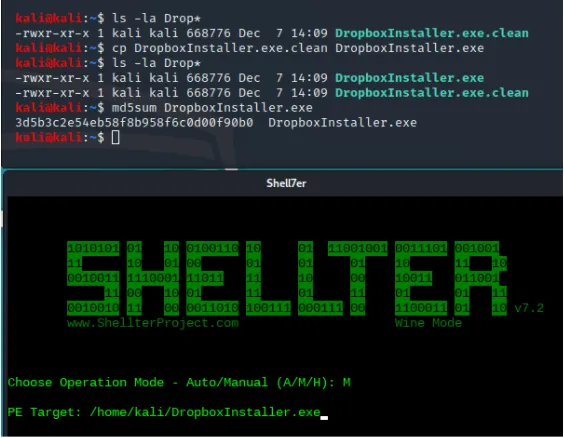# Shellter手动模式和自动模式的免杀对比

## 启动shellter：回答程序问题

shellter 立即开始提问。第一个是：是否应该包含动态线程上下文信息集合我们回答“不”。否则，此操作将减少可用注入点的数量。仅当您要仔细跟踪大文件时才启用此选项。该选项包括一个额外的过滤阶段。

## 跟踪后过滤和解码器混淆

``````import random random.seed() line=" "
for i in range(0,300) : r=random.randrange(4)
if r==0 and line[-1]!="x": line=line+"x"
elif r==1 and line[-1]!="!": line=line+"!"
elif r==2 and line[-1]!="-":
line=line+"+"
elif r==3 and line[-1]!="+": line=line+"-"``````

from